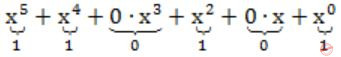Question 1

A bit-stuffing based framing protocol uses an 8-bit delimiter pattern of 01111110. If the output bit-string after stuffing is 01111100101, then the input bit-string is

 A 0111110100 B 0111110101 C 0111111101 D 0111111111
Question 1 Explanation:
Given 8-bit delimiter pattern of 01111110.
Output Bit string after stuffing is 01111100101.
 Question 2

Determine the maximum length of the cable (in km) for transmitting data at a rate of 500 Mbps in an Ethernet LAN with frames of size 10,000 bits. Assume the signal speed in the cable to be 2,00,000 km/s.

 A 1 B 2 C 2.5 D 5
Question 2 Explanation:
500×106 bits ------ 1 sec
∴104 bits -----5×108/104 = 104/5×108sec = 1/5×104sec
1 sec------2×105 km
1/5×104-----2×105/5×104 = 4 km
Maximum length of cable = 4/2 = 2 km
 Question 3

A channel has a bit rate of 4 kbps and one-way propagation delay of 20 ms. The channel uses stop and wait protocol. The transmission time of the acknowledgement frame is negligible. To get a channel efficiency of at least 50%, the minimum frame size should be

 A 80 bytes B 80 bits C 160 bytes D 160 bits
Question 3 Explanation:
 Question 4

In a TDM medium access control bus LAN, each station is assigned one time slot per cycle for transmission. Assume that the length of each time slot is the time to transmit 100 bits plus the end-to-end propagation delay. Assume a propagation speed of 2 × 108 m/sec. The length of the LAN is 1 km with a bandwidth of 10 Mbps. The maximum number of stations that can be allowed in the LAN so that the throughput of each station can be 2/3 Mbps is

 A 3 B 5 C 10 D 20
Question 4 Explanation:Question 5

Consider the following message M = 1010001101. The cyclic redundancy check (CRC) for this message using the divisor polynomial x5 + x4 + x2 + 1 is:

 A 01110 B 01011 C 10101 D 10110
Question 5 Explanation:
Degree of generator polynomial is 5. Hence, 5 zeroes is appended before division.
M = 1010001101append 5 zeroes = M = 101000110100000∴ CRC = 01110
 Question 6

Suppose that two parties A and B wish to setup a common secret key (D-H key) between themselves using the Diffie-Hellman key exchange technique. They agree on 7 as the modulus and 3 as the primitive root. Party A chooses 2 and party B chooses 5 as their respective secrets. Their D-H key is

 A 3 B 4 C 5 D 6
Question 6 Explanation:
For Diffe-Hellaman the secret key is (pab)modn,
where p is the primitive root and n is the modulus and 'a' and 'b' are the secret values selected by parity A & B.
32×5 mod 7 = 310 mod 7 = 4
 Question 7

Which one of the following statements is FALSE?

 A Packet switching leads to better utilization of bandwidth resources than circuit switching. B Packet switching results in less variation in delay than circuit switching. C Packet switching requires more per packet processing than circuit switching. D Packet switching can lead to reordering unlike in circuit switching.
 Question 8

Consider a simplified time slotted MAC protocol, where each host always has data to send and transmits with probability p = 0.2 in every slot. There is no backoff and one frame can be transmitted in one slot. If more than one host transmits in the same slot, then the transmissions are unsuccessful due to collision. What is the maximum number of hosts which this protocol can support, if each host has to be provided a minimum through put of 0.16 frames per time slot?

 A 1 B 2 C 3 D 4
Question 8 Explanation:
Let there be N such hosts. Then when one host is transmitting then others must be silent for successful transmission. So throughput per host,
0.16 = 0.2 × 0.8N-1
⇒ 0.8 = 0.8N-1
⇒ N = 2
 Question 9
Which of the following are Data link layer standard ?
(1) Ethernet
(2) HSSI
(3) Frame Relay
(4) 10base T
(5) Token ring
 A 1, 2 B 1, 3, 5 C 1, 3, 4, 5 D 1, 2, 3, 4, 5
Computer-Networks       Data-Link-Layer       UGC NET CS 2005 june-paper-2
Question 9 Explanation:
Except HSSI, all are belongs to data link layer.
The High-Speed Serial Interface (HSSI) is a differential ECL serial interface standard developed by Cisco Systems and T3plus Networking primarily for use in WAN router connections. It is capable of speeds up to 52 Mbit/s with cables up to 50 feet (15 m) in length.
 Question 10
The LAPB frame structure and the frame structure of SDLC are :
 A Opposite B Identical C Reversed D Non-identical
Computer-Networks       Data-Link-Layer       UGC NET CS 2006 Dec-paper-2
Question 10 Explanation:
Link Access Procedure, Balanced (LAPB) implements the data link layer as defined in the X.25 protocol suite. LAPB is a bit-oriented protocol derived from HDLC that ensures that frames are error free and in the correct sequence.
Frame format:*** The LAPB frame structure and the frame structure of SDLC are identical.
 Question 11
The design issue of Data link Layer in OSI Reference Model is
 A Framing B Representation of bits C Synchronization of bits D Connection control
Computer-Networks       Data-Link-Layer       UGC NET CS 2012 Dec-Paper-2
Question 11 Explanation:
Explanation:Question 12
Which of the following are Data Link Layer standard ?
1.Ethernet
2.HSSI
3.Frame Relay
4.10Base-T
5.Token Ring
 A 1, 2, 3 B 1, 3, 5 C 1, 3, 4, 5 D 1, 2, 3, 4, 5
Computer-Networks       Data-Link-Layer       UGC NET CS 2009 Dec-Paper-2
Question 12 Explanation:
→ HSSI is a physical layer standard.
→ Ethernet, Frame Relay, 10Base-T and Token Ring is using data link layer.
There are 12 questions to complete.